[BACK]
 Intelligent Automation & Soft ComputingDOI:10.32604/iasc.2022.025982Article

Adaptive Resource Allocation Neural Network-Based Mammogram Image Segmentation and Classification

Department of Electronics and Communication Engineering, Government College of Engineering, Salem, 636011, India
*Corresponding Author: G. Kavithaa. Email: kavi.dhanya@gmail.com
Received: 11 December 2021; Accepted: 18 January 2022

Abstract: Image processing innovations assume a significant part in diagnosing and distinguishing diseases and monitoring these diseases’ quality. In Medical Images, detection of breast cancer in its earlier stage is most important in this field. Because of the low contrast and uncertain design of the tumor cells in breast images, it is still challenging to classify breast tumors only by visual testing by the radiologists. Hence, improvement of computer-supported strategies has been introduced for breast cancer identification. This work presents an efficient computer-assisted method for breast cancer classification of digital mammograms using Adaptive Resource Allocation Network (ARAN). At first, breast cancer images were taken as input, preprocessing step is utilized to eliminate the noise and unimportant data from the image utilizing a Butterworth filter. Adaptive histogram equalization is utilized to improve the contrast of the image. Multimodal clustering segmentation has been applied, and Tetrolet transformation based feature extraction is applied at various levels, based on this, data classification is implemented. For exact classification, ARAN is utilized to predict if the patient is influenced by breast cancer. Compared with other current research techniques, the proposed strategy predicts the results efficiently. The overall accuracy of ARAN-based mammogram classification is 93.3%.

Keywords: Adaptive resource allocation neural network; butterworth filter; histogram equalization; breast cancer; mammogram; machine learning

1  Introduction

The leading cause of female deaths in the world is due to breast cancer. It has been observed that early detection of cancer can help to decrease the mortality rate among women, and it can potentially help to increase life expectancy. In breast cancer diagnosis, among the available various techniques, mammography is the most promising technique and used by radiologists frequently. Mammogram images are generally of low contrast and added with noise. On breast mammogram bright areas do not indicate cancer. In some mammograms, there may be malignant tissue and normal dense tissue.

Finding the difference in the contrast between malignant and normal dense tissues is not possible by applying manual identification processing. A mammogram is essential to understand cancerous lesions’ mass areas and understand the tumor and its segmentation. Therefore, the detection of malignant lesions in mammogram images is one of the active research areas. Many techniques, including computer-assisted detection systems and machine leaning-based methods, were introduced to segment breast cancer in mammogram images. However, no solution promises the best or can successfully meet the criteria of detecting only cancerous regions.

This work focuses on detecting tumors, representatives of the values of the more intense reference on the breast area. However, in special normal dense tissues with intensities similar to the tumor area, it is necessary to identify the tumor area, excluding these areas successfully. This work consists of four stages: Preprocessing, Segmentation, Feature extraction, and classification.

2  Literature Survey

Several researchers have been made to show an automatic recognition framework for the early analysis of breast cancer. In , authors used a Probabilistic Neural Network (PNN) based mammogram classification detailing a precision of 87.66%. In [4,5] proposed a Computer Aided Design (CAD) model using Grey Level Co-occurrence Matrix (GLCM) for feature extraction followed by order utilizing K-Nearest Neighbor (k-NN), Support Vector Machine (SVM) , and Artificial Neural Network (ANN) . Also, it consolidates histogram balance, some morphological tasks, and an Otsu’s-based thresholding strategy for dividing the Region of Interest (ROIs). As referenced, the classifiers’ exactness is 73%, 83%, and 77%, individually [8,9].

A mixture technique using wavelet and curvelet transform was introduced [10,11] to extract the mammograms’ features. In , they used a covering-based strategy for feature choice. This plan used the Fuzzy genetic framework for feature choice and obtained the precision of 89.47%. In , they proposed two distinctive automated techniques to order the classify the harmful mammogram tissues. The principal segmentation procedure is performed through an automated district developing whose ANN threshold is procured . The resulting segmentation is finished by a Convolutional Neural Network (CNN) whose limits are created utilizing a Genetic Algorithm (GA) [19,20].

At last, extraordinary characterization models like Multilayer Perceptron (MLP), k-NN, SVM, naıve Bayes, and arbitrary timberland are used, yielding a precision of 86.47% with MLP . In , they proposed a CAD framework to improve the extricated feature set utilizing the Glowworm Swarm Optimization (GSO) algorithm in mammograms . In their work, the features are acquired utilizing a GLCM. It is tracked down that all separated features are not important, and to moderate this, GSO is utilized to advance the Feature Vector (FV). Further, SVM is applied to characterize the mammogram as ordinary or unusual. This methodology yields an exactness of 87%.

3  ARAN with White Mass Estimation for Improved Micro Calcification Identification

Early detection of breast cancer is believed to increase survival. The best available breast imaging technique is to use low dose X-rays to detect breast cancer before experiencing mammography symptoms. Mammograms may indicate breast cancer in a better manner for the people with defects and micro calcifications. This research’s main objective is to develop image processing algorithms and increase the accuracy of breast cancer diagnostics in computer-assisted detection by categorizing women into different risk groups. The block diagram of the proposed method is shown in Fig. 1.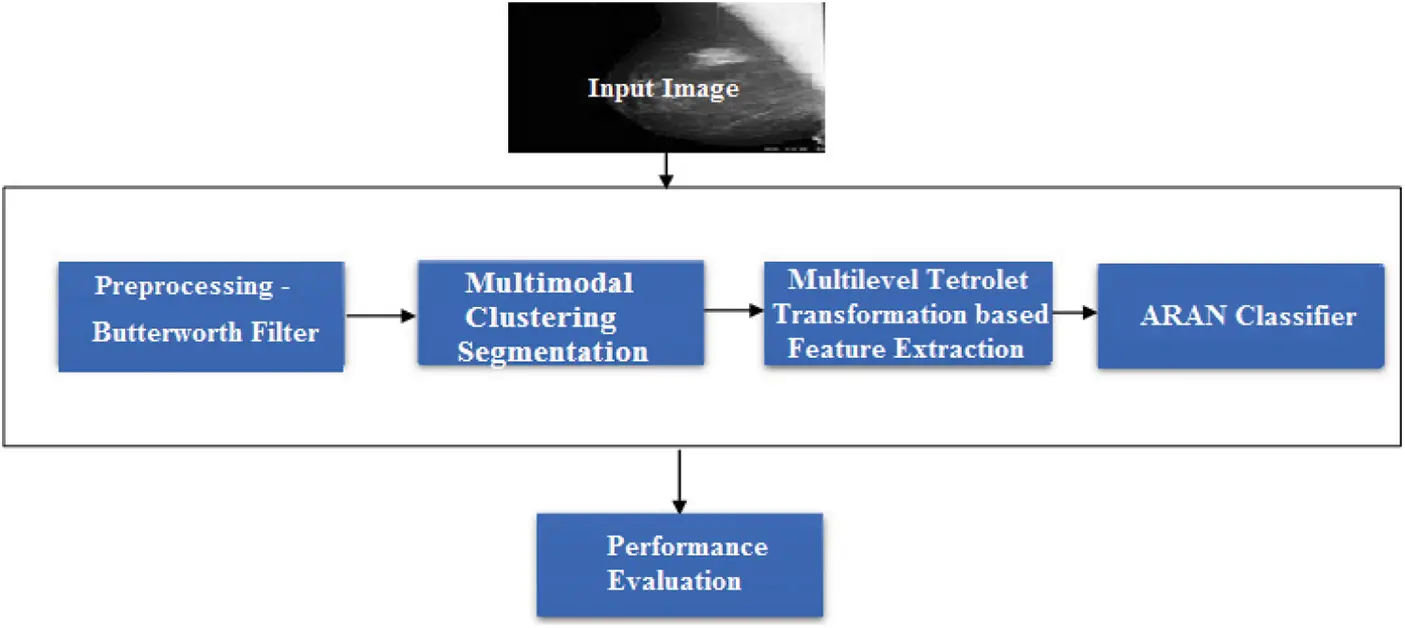Figure 1: Block diagram of the proposed system

3.1 Preprocessing –Butterworth Filter

Usually, digital mammogram images are distorted due to the sensors used and the other artifacts. Therefore, accurate results are not possible. So preprocessing techniques are used to improve images with higher accuracy results. In this work, a preprocessing method with a Butterworth filter is introduced to enhance the input image. The proposed preprocessing filter is tuned to the local ridgeline direction. The ridge frequency is applied to the channel pixel normalized input mammogram image to obtain an enhanced image.

The Result of the Preprocessing is shown in Fig. 2. As compared with the input image (Figs. 2a2c, 2g and 2h), the preprocessed (Figs. 2d2f, 2i and 2j) image have low noise.Figure 2: Input image (digital database for screening mammography (DDSM) dataset) and preprocessed image

3.2 Multimodal Clustering Segmentation

The second stage CAD-based mass detection plan, is to separate suspicious areas that include masses from the background parenchyma, i.e., divide the mammogram into several non-attempted areas and then exclude ROIs and place suspicious mass region. The suspect area is brighter than its surroundings and has an almost uniform density, the size is varied and has blurred boundaries. Segmenting the mass of a sculpture from others can be a complex process due to the diversity of the mass characteristics from one image to another. In this work, Multimodal clustering segmentation method is used. Multimodal Clustering Segmentation is considered the most important overlooked learning problem. This system finds and collects the unlabeled information. Clustering is the process of organizing objects into groups that are similar in some way to a member. A cluster is a set of items “similar” among them and “different” from other cluster objects. An iterative system starts relegating every pixel to the closest gathering center utilizing a different measure D (Eq. (3)), which consolidates separation of clustering accuracy (Eq. (4)) and separation of spatial accuracy (Eq. (5)).

D=(dcm)2+(dsS)2 (1)

dc=SpB(I(xi,yi,Sp)I(xj,yj,Sp))2 (2)

ds=(xijyij)2 (3)

where

D = Separation measure, dc = Clustering Accuracy

I = Number of Iterations, S = Quantity of separated Pixel

x = Set of Pixels, y = Set of cluster centers

In this work, the clustering result is formed from mammogram images for two classes. Each type has a three-dimensional vector character with a gray and black value per pixel along with the class label.

Input: Preprocessed image

Output: Number of Clusters

Begin

Step 1: Create and initialize a data structure

Step 2: Initialize and compute the centroid for each class

Cij=q=0miDati,j,qmi (4)

where Cij = centroid, j = number of features, q = number of patterns and i = total number of classes

Step 3: Calculate the data matrix in every pixel

3.1 obtain the result of centroid distance in every cluster.

Distance=(Pixel Data matrixCij )2 (5)

3.2 Calculate the minimum distance and Assign cluster labels

Pixeli=Classlabel(minimum(distance[i]) (6)

Step 4: Evaluate the objective function

J=i=1Nj=1Cμij|xiCj|2 (7)

where J is the objective function, N is the number of pixels in the image, C is the number of clusters, μ is the centroid of xi’s cluster, xi is the ith pixel in N, cj is jth cluster in C and |xi-cj| is the Euclidean distance between xi and c

Step 5: Move to step 3 and repeat the process until the objective function minimized

Step 6: label in the final class is assigned. The result of segmentation is shown in Fig. 3.Figure 3: Segmentation output

3.3 Multilevel Tetrolet Feature Extraction

The extracted feature distribution can specify each pixel of a digitized mammogram, according to their neighboring pixels. Feature extraction in mammogram analysis is practically inevitable, and a good selection of features gives it high accuracy. This feature values the image, which is called the feature vector helps in finding the abnormality. The Multilevel Tetrolet Transformation based Feature extraction method is used to extract the mammogram’s features in this work. In proposed approach, tetrolet transform is used for texture feature extraction. Raghuwanshi and Tyagi have proposed a new texture image retrieval system based on tetrolet transform. Tetrolet works on the principle of tetrominoes. One of the key advantages of using tetrolet is its adaptability. Since traditional wavelet transform works only in two directions while tetrolet analyzes the image according to local image geometry.

Algorithm of Multilevel Tetrolet Feature Extraction

Input: Gray scale image I of size N × N where N = 2k where k∈N , the number of decomposition level J (default value log2N1),number of covering num_cov=64.

Output: Texture Feature Vector

Initialization: Initialize database = null, r = 1 and count2 = 1.

Iterate as follows:

Step 1: Input image I, number of decomposition level J = 4, lowr-1 = I and num-cov = 64

Step 2: Apply the tetrolet transform to generate different frequency sub bands

lowj,h1j,h2j and h3j . Where low shows the low pass h1, h2 and h3 shows the high passes

for each block at jth sub band. //tetrolet decomposition and selection of best tile

Step 3: For each decomposition level r, where r < = J perform the following:

For count1 = 1 to 64

Separate one low and three high pass coefficients at each block.

Assign numbering using bijective mapping and store in database

Count = count1 + 1;

End

For count2 = 1 to 64

Select best tile among 64 at level r from database to get best local geometry of the image at each level of decomposition.

Selected best combination of low pass sub band = lowbr , where b is best selection at level r is used for further decomposition.

Count2 = count2 + 1;

End

r = r+1;

End

// feature vector creation

Step 4: For j = 1 to J

Calculate the standard deviation of lowbj,h1bj,h2bj and h3bj at each decomposition level where b is the best tile at level j for image geometry.

Calculate the energy lbj,h1bj,h2bj and h3bj .

Store standard deviation and energy in feature database for sub bands and level J. TFV{j}=[Standard deviation (j),Energy(j)] .

j = j+1;

End

Step5: output TFV

3.4 Adaptive Resource Allocation Neural Network Classifier

Adaptive Resource Allocation Network (ARAN) classifiers are trained feed-forward network type using supervised training algorithms. The ARAN network’s main advantage is that it uses only a single hidden layer and uses the radial basis function as its execution function. The ARAN network usually trains much faster than back-propagation systems. This network is less suspected of problems because of the behavior of the non-standard input in hidden units.

The Architecture of ARAN is shown in Fig. 4. The following Eq. (8) shows the overall network output of ARAN.

y(x)= i=1Mwie((|xci)22σ2) (8)

where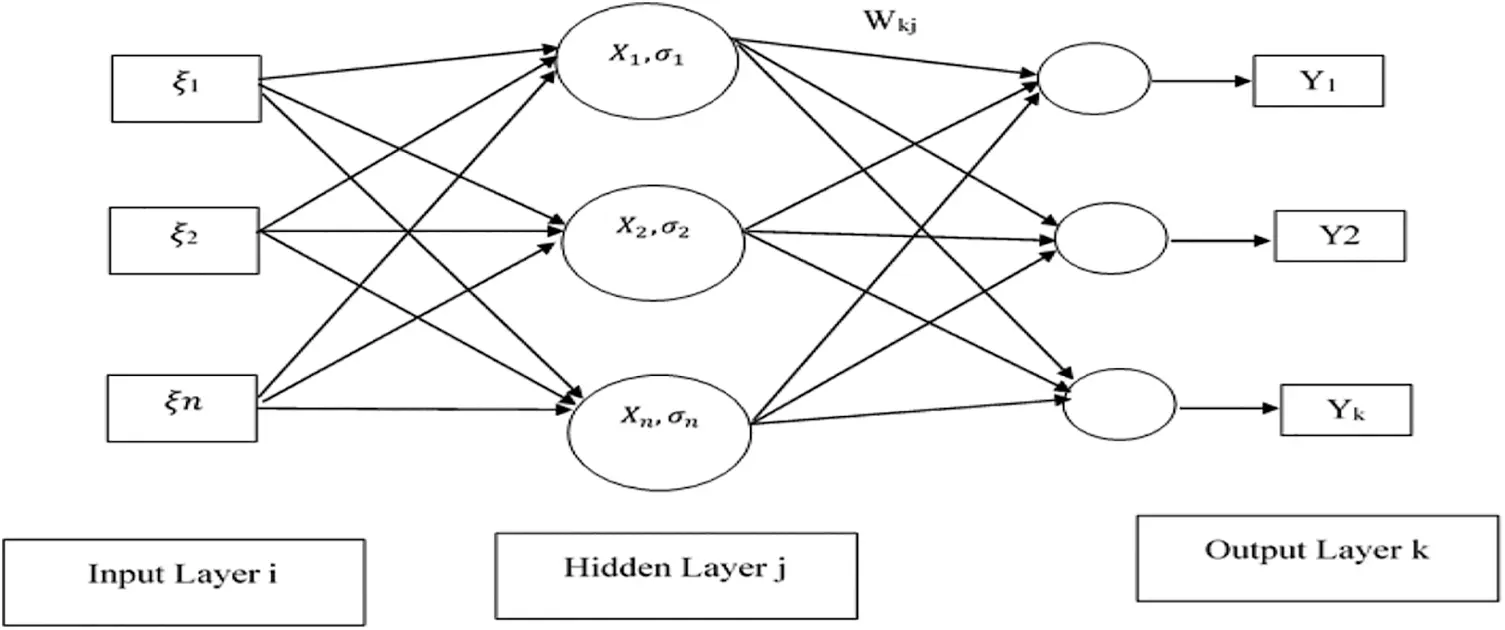Figure 4: Architecture of ARAN

x, y(x) = input and output function, ci = Cluster center

σ =  Center basis function, M = number of basis function centered.

Adaptive Resource Allocation Network is a type of sequential learning-based Radial Basis Function (RBF) network. Thus ARAN is a network that learning new computational units that allocate more patterns. The center (Xj) and a width (σj) are two important parameters associated with each hidden unit. The activation function of each hidden unit is symmetric to the input space. The output of every hidden unit depends only on the radial distance between the input vector ξi and the hidden unit Xj parameter center. The weight of every hidden unit and output unit are connected using Wkj.

The following equations discuss the overall network output.

Ok=jWkjVj , j=1 to n (number of hidden units) (9)

Vj=e|Xjξi|2 /2jσ2 (10)

where

Vj = jth hidden unit’s Result Wkj = Weight connect between hidden unit j and output k

Xj = Center σj = width of hidden unit

The learning period of ARAN includes the portion of new hidden units and the variation of network boundaries. The network starts with no hidden units, i.e., the network begins with no data’s and no patterns are yet stored. As input-output (ξi, yk) information are got during training, some of them are utilized for creating new hidden units relies upon the information which is chosen the accompanying conditions

d=|Xjξi|>δ (11)

e=|ykOk|>emin (12)

where

Xj = Center, δ and emin = Thresholds value of width and center

If the above two conditions are fulfilled, the information is viewed as unique, and another hidden unit is added. The principal level expresses that the information should be at a distance from all centers, and the subsequent condition expresses that the error between the network output and the target output must be significant. The emin addresses the ideal approximate accuracy of the network output, and the δ addresses the distance in resolution size of the input space. The network begins with δn = δmax. The estimation of δmax as the enormous interest in this info space, normally the whole non-resident input space is picked with zero probability. The distance δ is mathematically disintegrated as δ = max { δmax , γn, δmin }, where 0 < γ < 1 is the decay constant. The value for δ is decomposed until δmin, where it reaches the smallest length scale of interest. The exponential decaying of the norm allows far less basic tasks with larger widths. With the number of observations, the smaller widths’ functions are fine-tuned to separate the approximation. The following equations give the parameters with the new hidden unit.

Wkj(new)=e (13)

Xj(new)=Xj (14)

σj(new)=k|Xjξi| (15)

where

k = overlap factor determines the overlap of the hidden units’ responses in the input space. As k grows more extensive, the reactions of the units overlap more and more. When an observation (ξi, yk) is not pass new criteria, and a new hidden unit is not added. But the network parameters can only be adapted to match the Xj and Wjk observation. The Least Mean Squares (LMS) algorithm is used to adjusting the Xj and Wjk. The ARAN algorithm is discussed as follows

Algorithm of ARAN Network:

Step 1: Set δ=δmax

Step 2: For a given pair of input-output (ξi, yk), Compute the output Ok=jWkjVj , j=1 to n

Step3: Compute the error e = yk − Ok

Step 4: Compute δ = max { δmax , γn, δmin },

Step 5: If d > δ and e > emin, then insert a Radial Bias Function unit into the hidden layer,

set its width σj = k|Xj − ξi| and set the center coordinates equal to

input pattern

K = overlap factor that determines the amount of overlap of the

responses of the hidden units in the input space

Else

Step 6: Update the weights using the following equations

Wkj(new) = Wkj(old) + α * e * Vj Where α is learning Rate

Xji(new)=Xij(old)+ΔXji

where

ΔXji=2ησ2(Xjξi)Vj(Okyk)Wkj

Step 7: Save the network parameters and classify the result of mammogram

The overall flow chart of the proposed ARAN is shown in Fig. 5. Accessed from the exclusive image features, this method can distinguish pixels that respect themselves on a low resolution scale. The technology selects a small pixel arrangement with the micro calcification to determine the pixel that exceeds the clear edge self-esteem light. Based on the number of distinguished pixels and the area’s span, the proposed system dealing with micro calcification depth measures. The comparability of small-scale calcifications is registered by the similarity of the more in-depth actions of smaller-scale calcifications. Each segment has its small-scale calcification depth measure given the deep micro calcification measures, the comparability with other areas in this work process.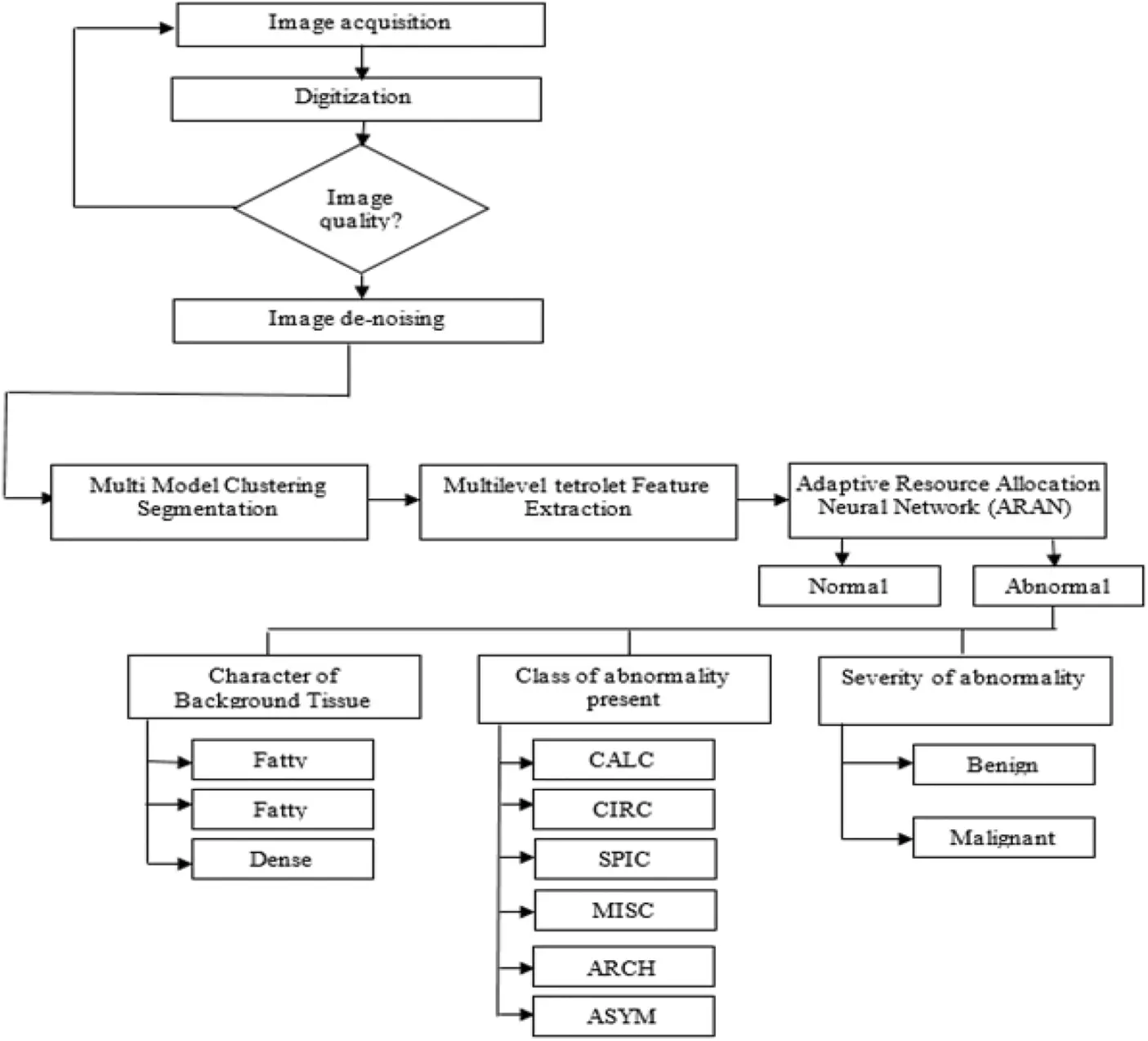Figure 5: Flow chart of proposed ARAN based mammogram classification

4  Experimental Validations

This section discusses the simulation results and performance analysis of the proposed Adaptive Resource Allocation Neural Network-based mammogram classification system. For evaluation, the Digital Database for Screening Mammography (DDSM) and Mammographic Image Analysis Society (MIAS) data set have been used. The details of datasets are shown in Tab. 1, and the Graphical User Interface (GUI) screen of the proposed system is shown in Fig. 6. The proposed model is simulated using MATLAB software.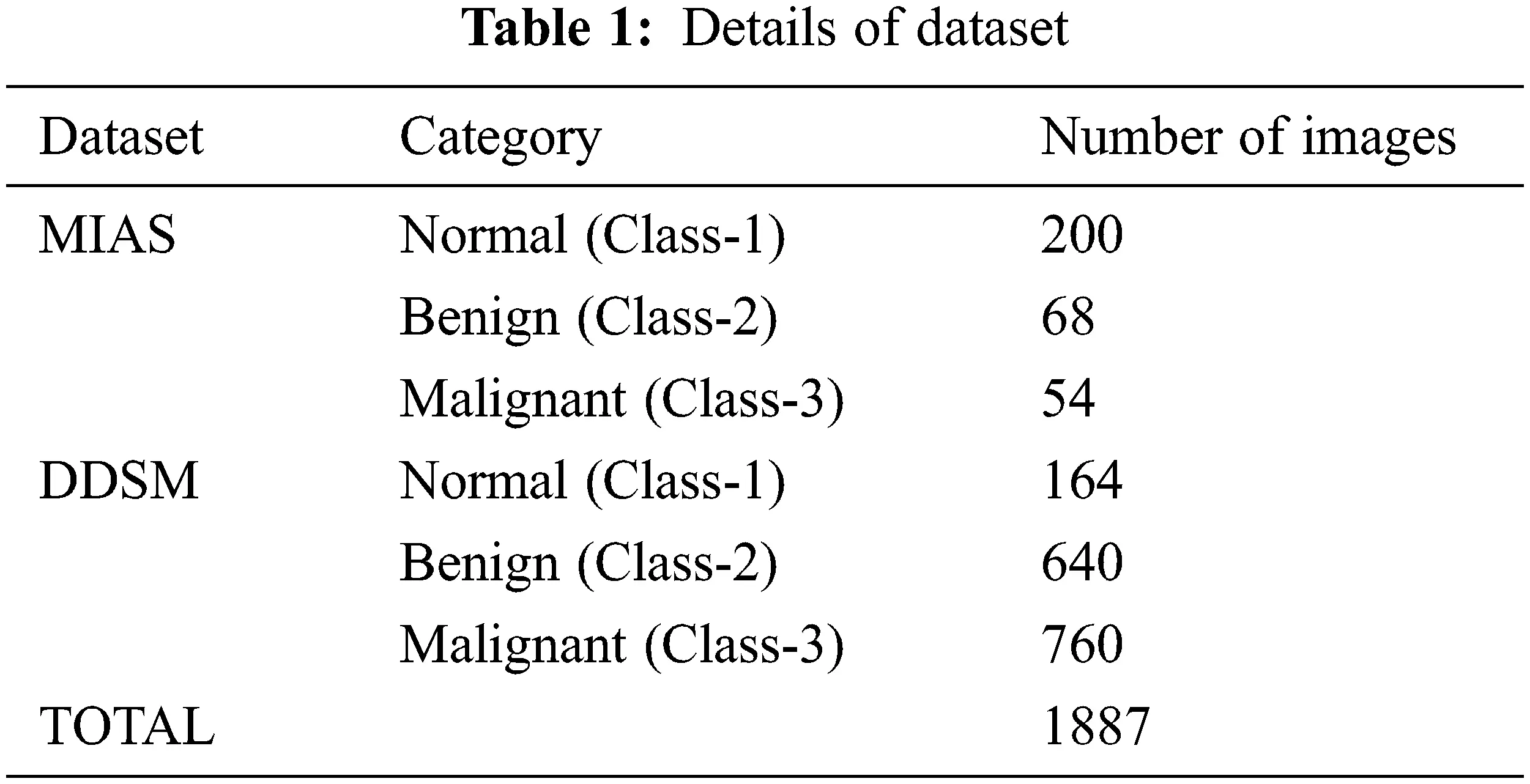Figure 6: GUI screen of proposed system

The simulation result screenshot of input data training and error ratio evaluation of the proposed Adaptive Resource Allocation Neural network-based mammogram classification system is shown in Fig. 7. The simulation result screenshot of input data training weight adjustment of proposed adaptive resource allocation neural network-based mammogram classification system is shown in Fig. 8. The simulation result screenshot of input test data with weight adjustment of proposed adaptive resource allocation neural network-based mammogram classification system is shown in Fig. 9.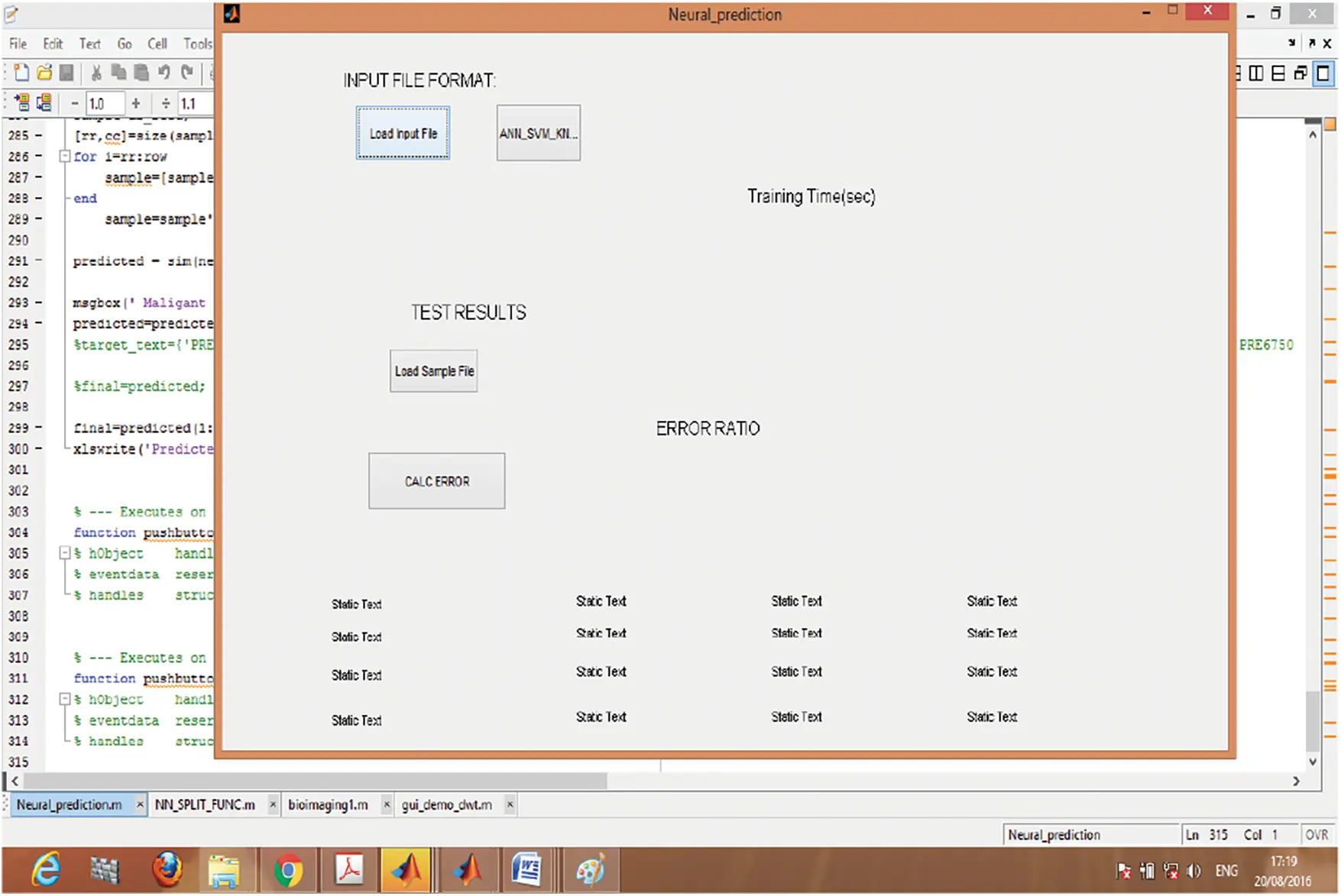Figure 7: Input data training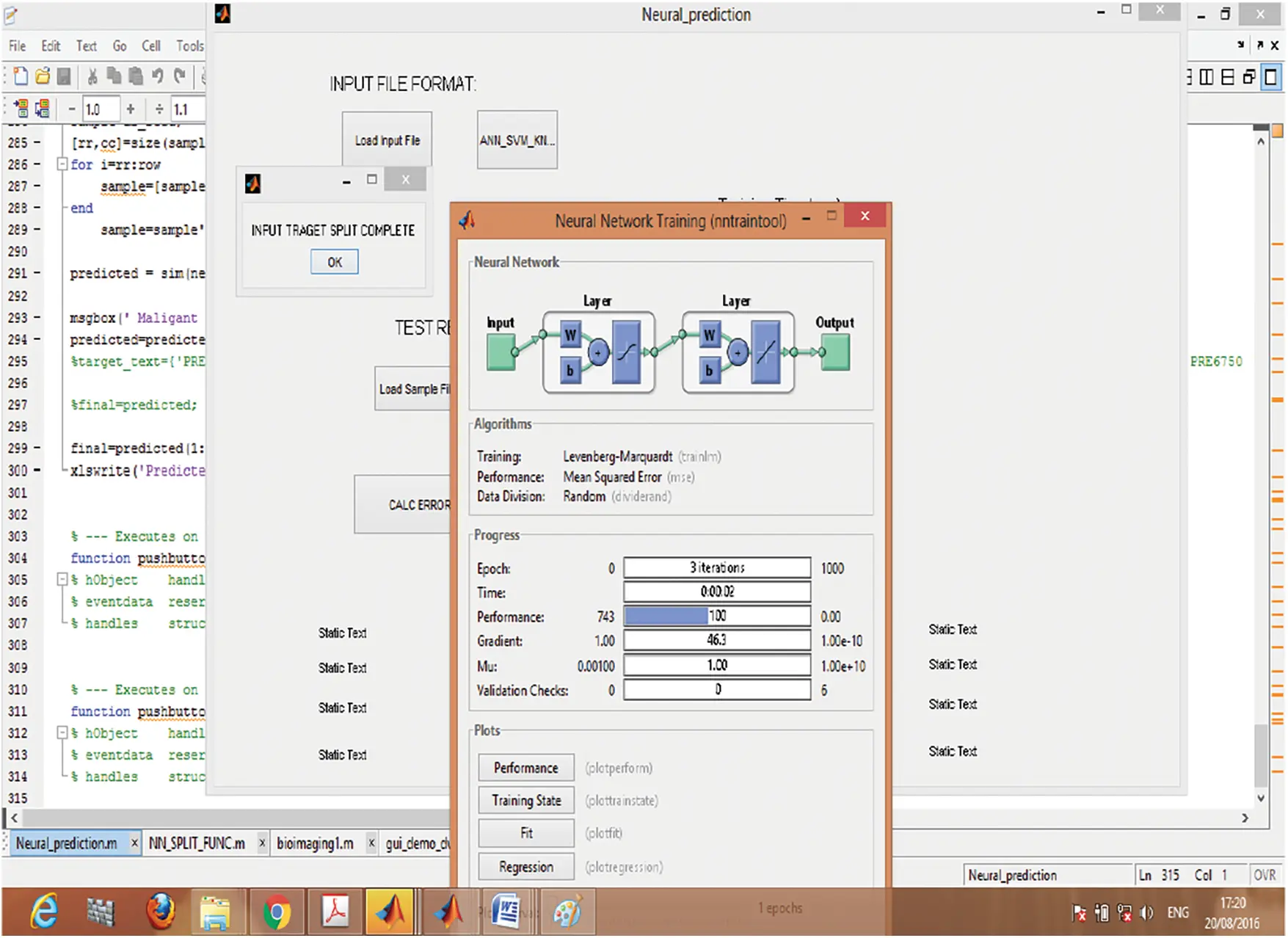Figure 8: Simulation result of weight adjustment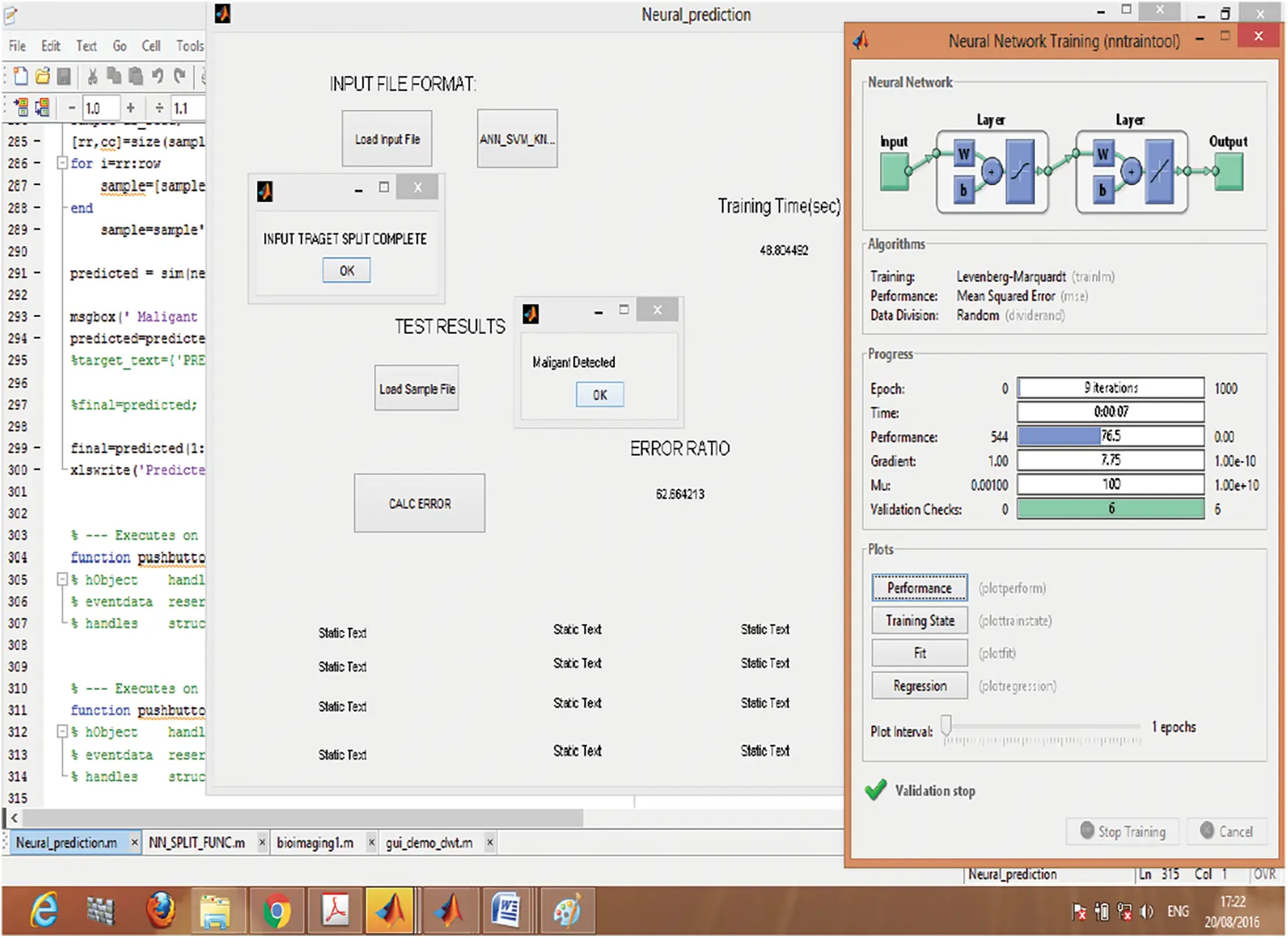Figure 9: GUI screen of testing

The simulation result screenshot of abnormality classification of the proposed adaptive resource allocation neural network-based mammogram classification system is shown in Fig. 10. The following Class of abnormality is present: Calcification (CALC), Well-defined/circumscribed masses (CIRC), Spiculated masses (SPIC), ill-defined masses (MISC), Architectural distortion (ARCH), Asymmetry (ASYM) and Normal (NORM).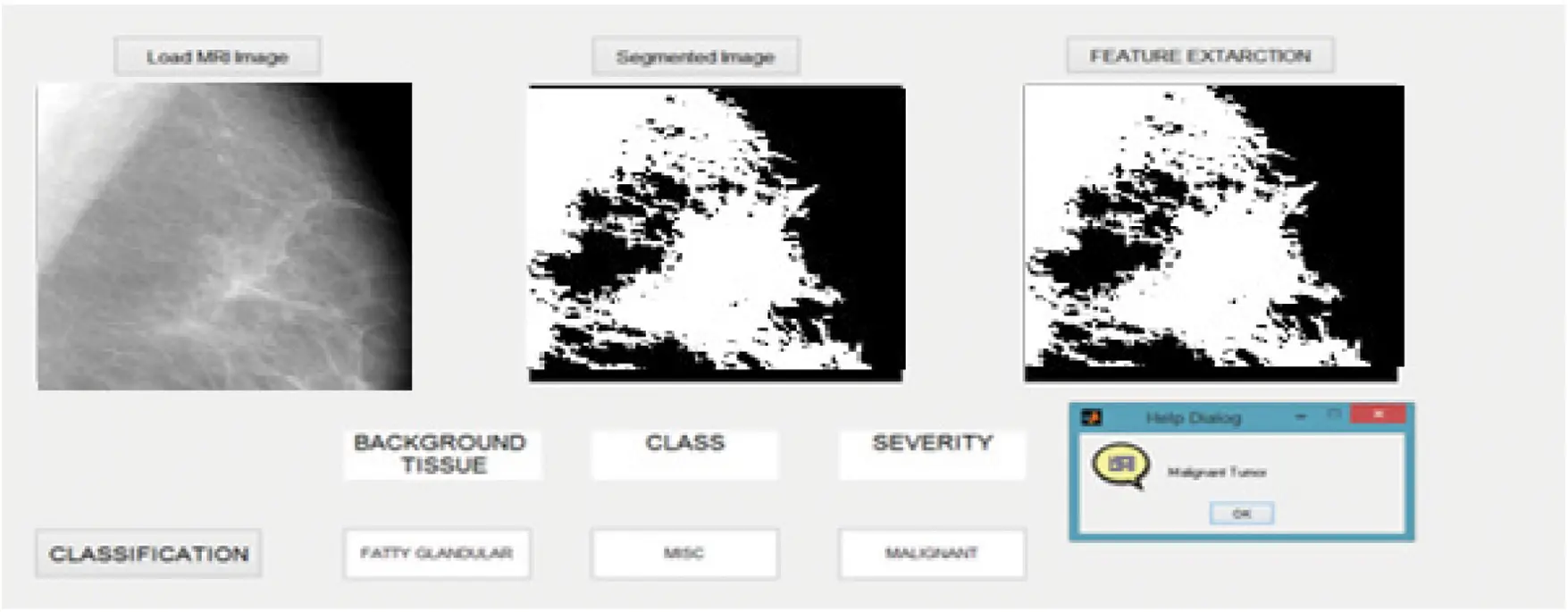Figure 10: Simulation result with abnormality classification

Tabs. 2 and 3 discuss the confusion matrix’s simulation results for class-1 in MIAS and DDSM datasets with Adaptive Resource allocation neural networks. The result of this class-1 confusion matrix is used to evaluate the performance of the proposed system.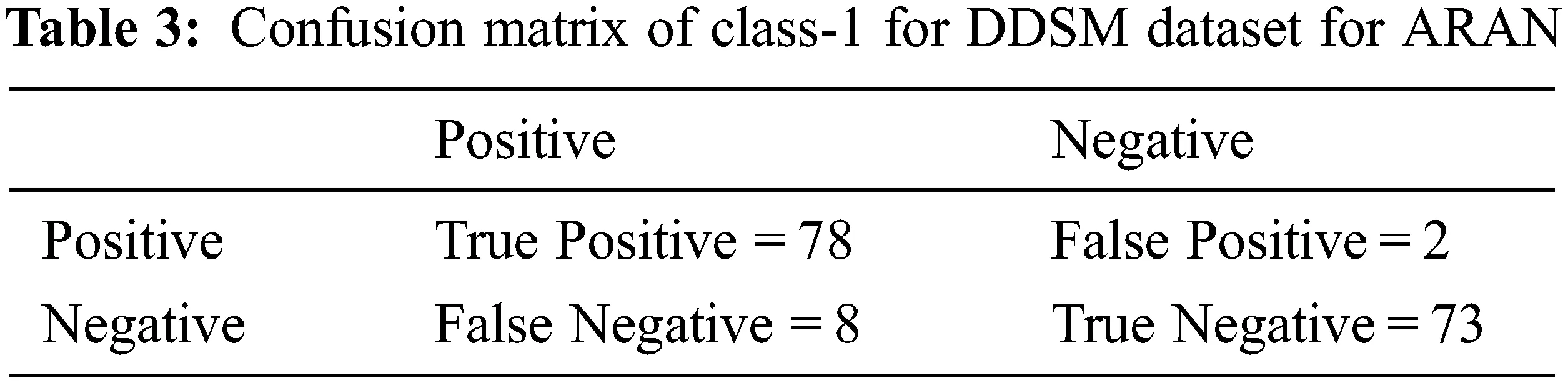The classification result of class-1 for the MIAS and DDSM dataset is shown in Fig. 11. The proposed ARAN classifier perfectly classify the result of class-1. The sensitivity, specificity and accuracy of Proposed ARAN with MIAS dataset’s class-1 results are 92.45%, 97.87% and 95.0%, respectively. The sensitivity, specificity and accuracy of Proposed ARAN with the DDSM dataset’s class-1 results are 90.69%, 97.33% and 93.78%, respectively.Figure 11: Classification result of class-1

Tabs. 4 and 5 discuss the confusion matrix’s simulation results for class-2 in MIAS and DDSM datasets, respectively. The result of this class-2 confusion matrix is used to evaluate the performance of the proposed system as shown in Fig. 12.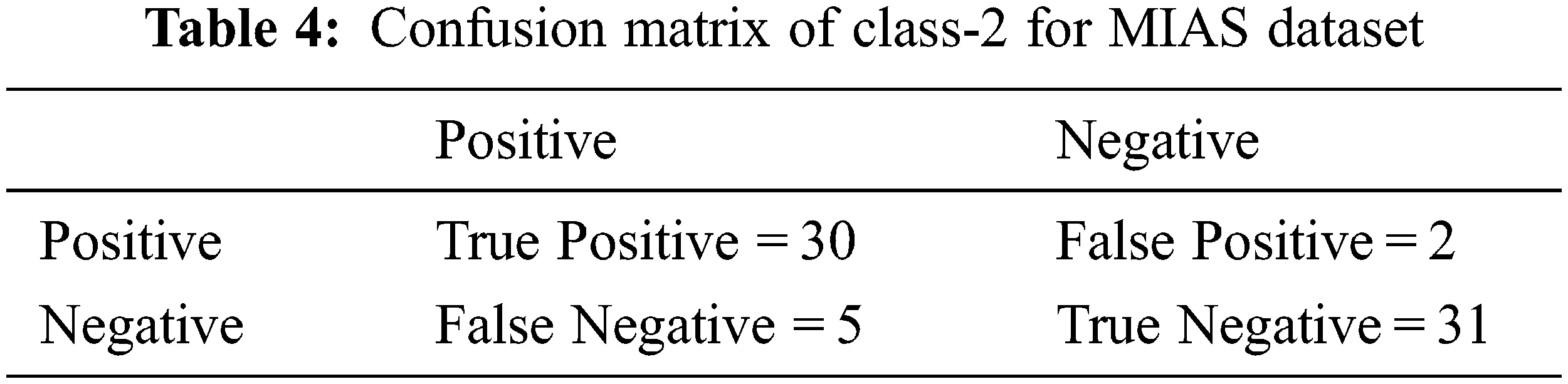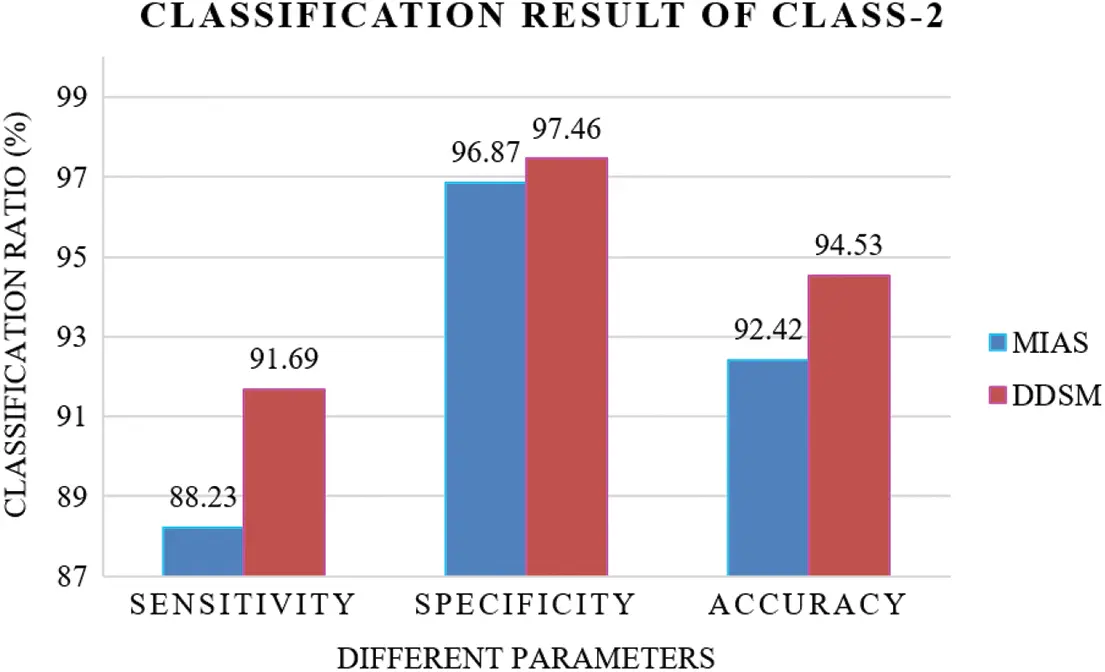Figure 12: Classification result of class-2

Tabs. 6 and 7 discuss the confusion matrix’s simulation results for class-3 in MIAS and DDSM datasets. The result of this class-3 confusion matrix is used to evaluate the performance of the proposed system.The classification result of class-3 for the MIAS and DDSM dataset is shown in Fig. 13. The proposed ARAN classifier perfectly classify the result of class-3. The sensitivity, specificity and accuracy of Proposed ARAN with MIAS dataset’s class-3 results are 89.65%, 96.0% and 92.59%. The sensitivity, specificity and accuracy of Proposed ARAN with DDSM dataset’s class-3 results are 91.86%, 96.30% and 94.07%, respectively.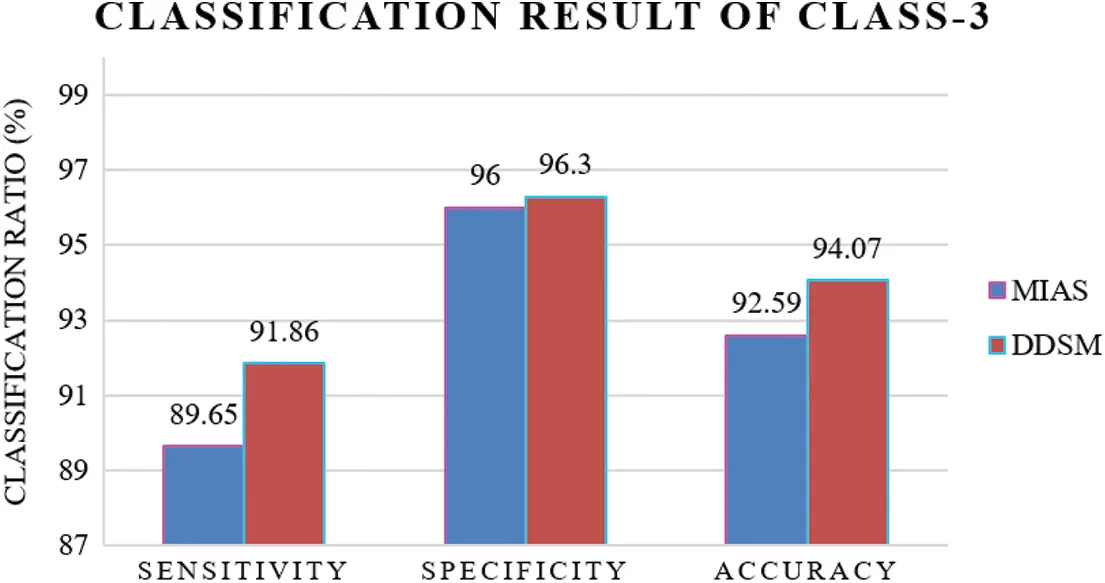Figure 13: Classification result of class-3

Tab. 8 discusses the proposed ARAN method’s overall classification ratio analysis with some other existing processes with the MIAS dataset. As compared with existing SVM and PCA methods, the proposed methods achieve the best classification accuracy. The overall accuracy of ARAN-based mammogram classification is 93.33%.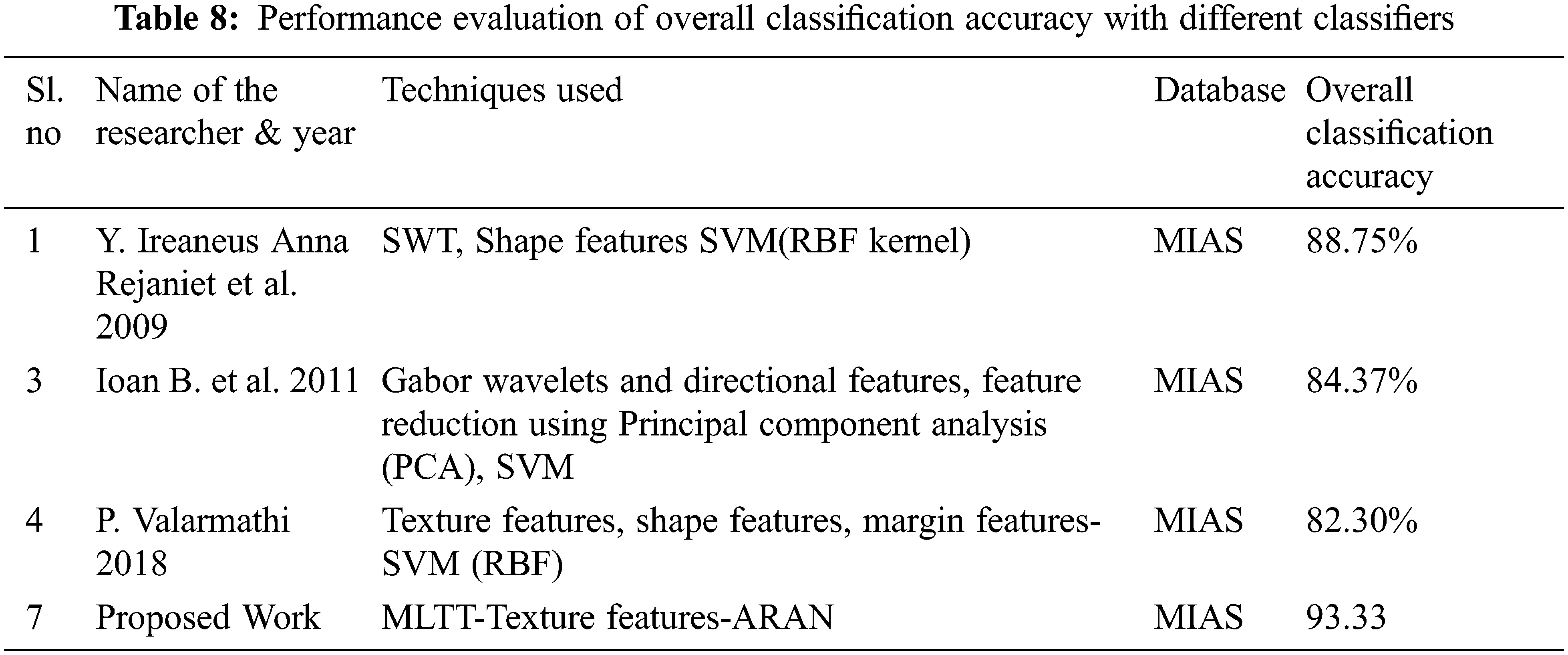Fig. 14 discusses the overall classification ratio accuracy analysis of the proposed method with some other existing methods. As compared with existing methods, the proposed ARAN gives the best result against the MIAS dataset. The overall accuracy of ARAN-based mammogram classification is 93.33%.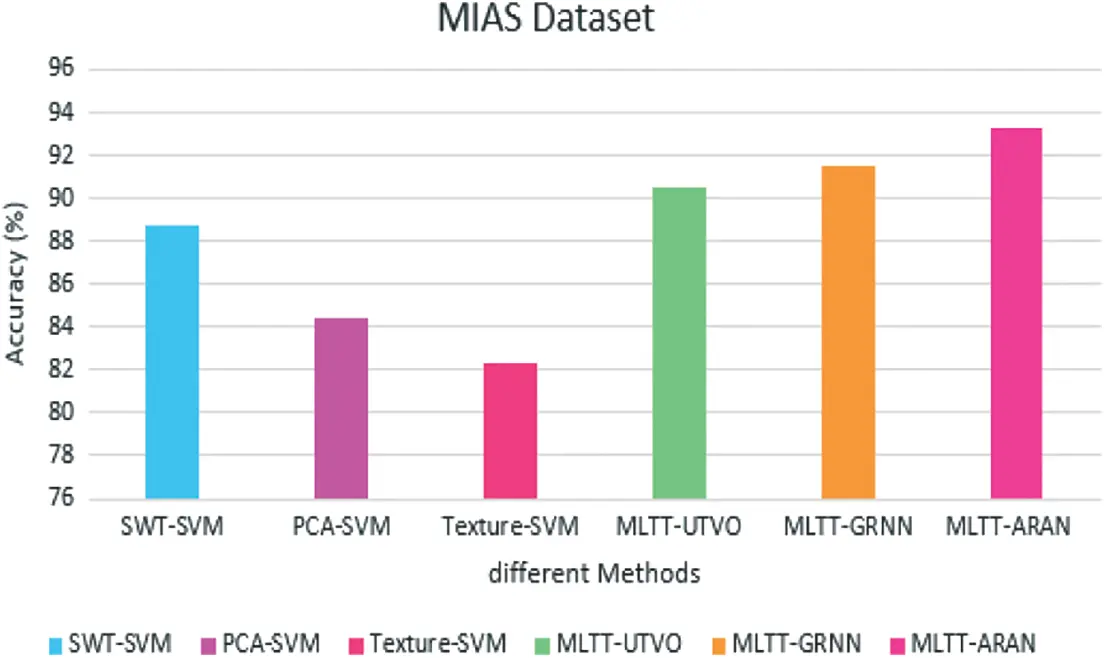Figure 14: Overall classification ratio analysis-ARAN

5  Conclusion

This work demonstrates how neural networks are used to detect, segment, and classify mammograms being expressed. Using the centers and widths of the hidden nodes as templates for detection and segmentation provides a guide for intelligent search through possible mammogram space. This approach increases cancer detection by collecting the desired specific objects to submit a request; improving segmentation by generating high-quality initial outlines with different objects. The centers and widths are relatively new using the Adaptive Resource Allocation Neural Network to creating a tight set and well-separated clusters. The system supports a clear screen hidden in nodes as templates. The simulation results demonstrate that the proposed method’s learning rate is good. The overall sensitivity, specificity and accuracy of the MIAS-based dataset are 90.11%, 96.91% and 93.33%. The overall sensitivity, specificity and accuracy of DDSM based dataset are 91.41%, 97.03% and 94.10%, respectively. Further directions of this work shall include a deep learning method to improve the performance and reduce the computational complexity.

Funding Statement: The authors received no specific funding for this study.

Conflicts of Interest: The authors declare that they have no conflicts of interest to report regarding the present study.

## References

1. Y. Zhao, D. Chen, H. Xie, S. Zhang and L. Gu, “Mammographic image classification system via active learning,” Journal of Medical and Biological Engineering, vol. 39, no. 4, pp. 569–582, 2019.
2. A. Voulodimos, N. Doulamis, A. Doulamis and E. Protopapadakis, “Deep learning for computer vision: A brief review,” Computational Intelligence and Neuroscience, vol. 2018, pp. 1–13, 2018.
3. X. Yu, Z. Yu, L. Wu, W. Pang and C. Lin, “Data-driven two-layer visual dictionary structure learning,” Journal of Electronic Imaging, vol. 28, no. 2, pp. 1, 2019.
4. X. Yu, W. Pang, Q. Xu and M. Liang, “Mammographic image classification with deep fusion learning,” Scientific Reports, vol. 10, no. 1, pp. 14361, 2020.
5. X. Yu, Z. Zhang, L. Wu, W. Pang, H. Chen et al., “Deep ensemble learning for human action recognition in still images,” Complexity, vol. 2020, pp. 1–23, 2020.
6. G. Yang, N. Xu and F. Li, “Research on deep learning classification for nonlinear activation function,” Journal of Jiangxi University of Science and Technology, vol. 39, pp. 76–83, 2018.
7. J. Wang, X. Yang, H. Cai, W. Tan, C. Jin et al., “Discrimination of breast cancer with microcalcifications on mammography by deep learning,” Scientific Reports, vol. 6, no. 1, pp. 27327, 2016.
8. B. Q. Huynh, H. Li and M. L. Giger, “Digital mammographic tumor classification using transfer learning from deep convolutional neural networks,” Journal of Medical Imaging, vol. 3, no. 3, pp. 034501, 2016.
9. B. Li, Y. Ge, Y. Zhao, E. Guan and W. Yan, “Benign and malignant mammographic image classifcation based on convolutional neural networks,” in Proc. of the 2018 10th Int. Conf. on Machine Learning and Computing, Macau, China, pp. 247–251, 2018.
10. D. Lévy and A. Jain, “Breast mass classification from mammograms using deep convolutional neural networks,” arXiv preprint arXiv :1612.00542, 2016.
11. H. Cai, Q. Huang, W. Rong, Y. Song, J. Li et al., “Breast microcalcification diagnosis using deep convolutional neural network from digital mammograms,” Computational and Mathematical Methods in Medicine, vol. 2019, pp. 1–10, 2019.
12. L. V. D. Maaten and G. Hinton, “Visualizing data using t-sne,” Journal of Machine Learning Research, vol. 9, pp. 2579–2605, 2008.
13. S. Jenifer, S. Parasuraman and A. Kadirvelu, “Contrast enhancement and brightness preserving of digital mammograms using fuzzy clipped contrast-limited adaptive histogram equalization algorithm,” Applied Soft Computing, vol. 42, pp. 167–177, 2016.
14. K. Simonyan and A. Zisserman, “Very deep convolutional networks for large-scale image recognition,” arXiv preprint arXiv:1409.1556, 20
15. C. Szegedy, W. Liu, Y. Jia, P. Sermanet, S. Reed et al., “Going deeper with convolutions,” in 2015 IEEE Conf. on Computer Vision and Pattern Recognition (CVPR), Boston, MA, pp. 1–9, 20
16. P. K. Upadhyay and S. Chandra, “Salient bag of feature for skin lesion recognition,” International Journal of Performability Engineering, vol. 15, pp. 1083–1093, 2019.
17. J. B. Huang and M. H. Yang, “Fast sparse representation with prototypes,” in 2010 IEEE Computer Society Conf. on Computer Vision and Pattern Recognition, San Francisco, CA, USA, pp. 3618–3625, 2010.
18. Y. Liu, X. Chen, R. K. Ward and Z. J. Wang, “Image fusion with convolutional sparse representation,” IEEE Signal Processing Letters, vol. 23, no. 12, pp. 1882–1886, 2016.
19. S. Khan, M. Hussain, H. Aboalsamh and G. Bebis, “A comparison of different Gabor feature extraction approaches for mass classification in mammography,” Multimedia Tools and Applications, vol. 76, no. 1, pp. 33–57, 2017.
20. G. Huang, Z. Liu, L. V. D. Maaten and K. Q. Weinberger, “Densely connected convolutional networks,” in Proc. of the IEEE Conf. on Computer Vision and Pattern Recognition, Honolulu, HI, USA, pp. 4700–4708, 2017.
21. V. K. Singh, S. Romani, H. A. Rashwan, F. Akram, N. Pandey et al., “Conditional generative adversarial and convolutional networks for X-ray breast mass segmentation and shape classification,” in Proc. of the Medical Image Computing and Computer Assisted Intervention; MICCAI’18, Granada, Spain, pp. 833–840, 2018.
22. V. Agarwal and C. Carson, “Using deep convolutional neural networks to predict semantic features of lesions in mammograms,” C231n Course Project Reports, 2015.
23. F. Gao, T. Wu, J. Li, B. Zheng, L. Ruan et al., “SD-CNN: A shallow-deep CNN for improved breast cancer diagnosis,” Computerized Medical Imaging and Graphics, vol. 70, pp. 53–62, 2018.
24. Y. B. Hagos, A. G. Mérida and J. Teuwen, “Improving breast cancer detection using symmetry information with deep learning,” in Proc. of the Image Analysis for Moving Organ, Breast, and Thoracic Images; RAMBO’18, Granada, Spain, pp. 90–97, 2018.
25. G. Valvano, G. Santini, N. Martini, A. Ripoli, C. Iacconi et al., “Convolutional neural networks for the segmentation of microcalcification in mammography imaging,” Journal of Healthcare Engineering, vol. 2019, pp. 1–9, 2019.This work is licensed under a Creative Commons Attribution 4.0 International License, which permits unrestricted use, distribution, and reproduction in any medium, provided the original work is properly cited.